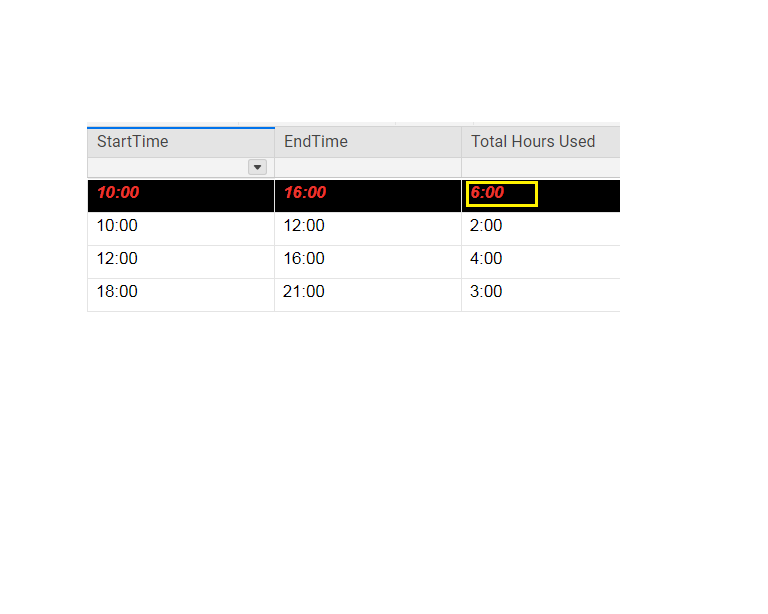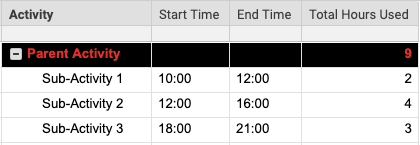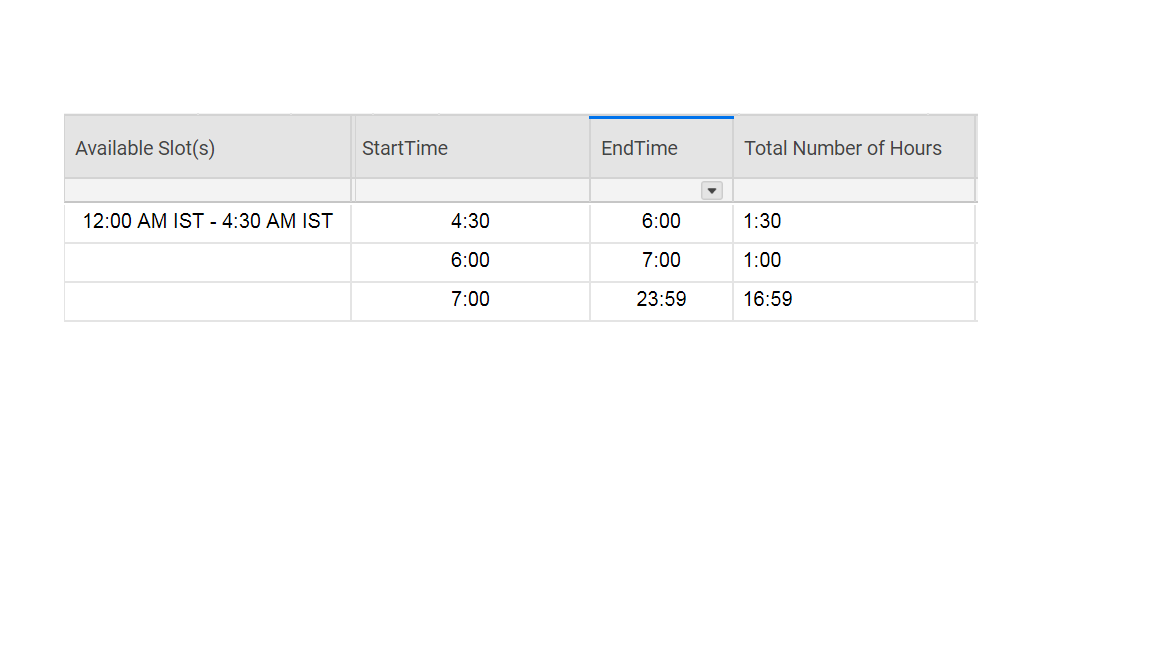# Calculating Sum of Hours from Child Rows

I am trying to sum up the "Total Hours Used" from Child rows (white highlighted) into the "Total Hours Used" parent row (black highlighted). I cannot use StartTime and EndTime here, because the time slots are not necessarily continuous. Appreciate any inputs on this.

Child Rows - "Total Hours Used" Calculated as 2 hours, 4 hours and 3 hours respectively.

Parent Row - Expected "Total Hours Used" = 2:00+4:00+3:00=9:00.

I checked a bunch of threads, but I wasn't able to find a solution for this one. Any inputs would be highly appreciated.• Will Start and End always be the same day, and will it always be hours without minutes?

• Are you using indenting within your sheet to create the Parent and Child rows?  If so, then you can use the formula in the Parent row:

=SUM(CHILDREN())

This would sum up Total Hours Used based on white background rows being children of the black background row.

Note that time arithmetic is challenging in Smartsheet (for me anyway!).  So I'm not sure how to calculate the number of hours on each row.  But hopefully this shows what I mean with the SUM(CHILDREN()) functions.• @Jeremy

This is a good thought, but it will only work if the Total Hours Used is in a numerical value. When the Total Hours Used is in hh:mm format, it is a text string and therefore cannot be summed.

Calculating the difference between start and end times will eventually lead to this numerical value which in turn can then be summed and then converted back into the hh:mm format for display.

This step of SUM(CHILDREN()) would eventually be used, but not until closer to the end after all of the other calculations have been completed.

.

@dpaul

The reasoning behind my two initial questions is that certain aspects can increase the complexity of the calculations. It is all able to be done regardless of how you answer the questions, but there's no reason to factor in different dates and 12 vs. 24 hour times if they won't be an issue.

• "Note that time arithmetic is challenging in Smartsheet (for me anyway!).  So I'm not sure how to calculate the number of hours on each row."

.

If you'd like a few pointers on how to "simplify" things to make time calculation workarounds a little easier (or at least a little more understandable), I'd be more than happy to help.

But....

If you don't need to do time calculations anytime soon, there are rumors that SS is working on rolling out some type of way to do this much more efficiently (fingers crossed) sometime this year.

• Thanks Paul, Jeremy. Apologies for the delay in getting back.

1. Will Start and End always be the same day, and will it always be hours without minutes? It will always be within the same day for this use case. I found this formula for calculation of difference in time and this works just fine - it gives me the results as HH:MM

=INT((((IF(LEFT(EndTime2, FIND(":", EndTime2) - 1) = "12", IF(OR(FIND("a", EndTime2) > 0, FIND("p", EndTime2) > 0), 0, 12), VALUE(LEFT(EndTime2, FIND(":", EndTime2) - 1))) + IF(FIND("p", EndTime2) > 0, 12)) * 60 + VALUE(MID(EndTime2, FIND(":", EndTime2) + 1, 2))) - ((IF(LEFT(StartTime2, FIND(":", StartTime2) - 1) = "12", IF(OR(FIND("a", StartTime2) > 0, FIND("p", StartTime2) > 0), 0, 12), VALUE(LEFT(StartTime2, FIND(":", StartTime2) - 1))) + IF(FIND("p", StartTime2) > 0, 12)) * 60 + VALUE(MID(StartTime2, FIND(":", StartTime2) + 1, 2)))) / 60) + ":" + IF(((((IF(LEFT(EndTime2, FIND(":", EndTime2) - 1) = "12", IF(OR(FIND("a", EndTime2) > 0, FIND("p", EndTime2) > 0), 0, 12), VALUE(LEFT(EndTime2, FIND(":", EndTime2) - 1))) + IF(FIND("p", EndTime2) > 0, 12)) * 60 + VALUE(MID(EndTime2, FIND(":", EndTime2) + 1, 2))) - ((IF(LEFT(StartTime2, FIND(":", StartTime2) - 1) = "12", IF(OR(FIND("a", StartTime2) > 0, FIND("p", StartTime2) > 0), 0, 12), VALUE(LEFT(StartTime2, FIND(":", StartTime2) - 1))) + IF(FIND("p", StartTime2) > 0, 12)) * 60 + VALUE(MID(StartTime2, FIND(":", StartTime2) + 1, 2)))) - INT((((IF(LEFT(EndTime2, FIND(":", EndTime2) - 1) = "12", IF(OR(FIND("a", EndTime2) > 0, FIND("p", EndTime2) > 0), 0, 12), VALUE(LEFT(EndTime2, FIND(":", EndTime2) - 1))) + IF(FIND("p", EndTime2) > 0, 12)) * 60 + VALUE(MID(EndTime2, FIND(":", EndTime2) + 1, 2))) - ((IF(LEFT(StartTime2, FIND(":", StartTime2) - 1) = "12", IF(OR(FIND("a", StartTime2) > 0, FIND("p", StartTime2) > 0), 0, 12), VALUE(LEFT(StartTime2, FIND(":", StartTime2) - 1))) + IF(FIND("p", StartTime2) > 0, 12)) * 60 + VALUE(MID(StartTime2, FIND(":", StartTime2) + 1, 2)))) / 60) * 60) < 10, "0") + ((((IF(LEFT(EndTime2, FIND(":", EndTime2) - 1) = "12", IF(OR(FIND("a", EndTime2) > 0, FIND("p", EndTime2) > 0), 0, 12), VALUE(LEFT(EndTime2, FIND(":", EndTime2) - 1))) + IF(FIND("p", EndTime2) > 0, 12)) * 60 + VALUE(MID(EndTime2, FIND(":", EndTime2) + 1, 2))) - ((IF(LEFT(StartTime2, FIND(":", StartTime2) - 1) = "12", IF(OR(FIND("a", StartTime2) > 0, FIND("p", StartTime2) > 0), 0, 12), VALUE(LEFT(StartTime2, FIND(":", StartTime2) - 1))) + IF(FIND("p", StartTime2) > 0, 12)) * 60 + VALUE(MID(StartTime2, FIND(":", StartTime2) + 1, 2)))) - INT((((IF(LEFT(EndTime2, FIND(":", EndTime2) - 1) = "12", IF(OR(FIND("a", EndTime2) > 0, FIND("p", EndTime2) > 0), 0, 12), VALUE(LEFT(EndTime2, FIND(":", EndTime2) - 1))) + IF(FIND("p", EndTime2) > 0, 12)) * 60 + VALUE(MID(EndTime2, FIND(":", EndTime2) + 1, 2))) - ((IF(LEFT(StartTime2, FIND(":", StartTime2) - 1) = "12", IF(OR(FIND("a", StartTime2) > 0, FIND("p", StartTime2) > 0), 0, 12), VALUE(LEFT(StartTime2, FIND(":", StartTime2) - 1))) + IF(FIND("p", StartTime2) > 0, 12)) * 60 + VALUE(MID(StartTime2, FIND(":", StartTime2) + 1, 2)))) / 60) * 60)

2. Are you using indenting within your sheet to create the Parent and Child rows?  If so, then you can use the formula in the Parent row:

I just added indenting as per your suggestion, however am not sure how to use Sum (Children()). I tried =SUM(CHILDREN([Total Number of Hours])) but it gives me an error "Unparseable"

3. As of now, I am only able to get the total hours to sum up to 23:59 and not 24 hours. Is there a way to get 24 hours?

Eventually, I need to know how many hours out of 24 hours is a system being utilized - i.e. calculate it's available capacity. It would also be great if SS is able to give me "available time slots" as depicted in the screenshot.

Let me know if you have any more questions.